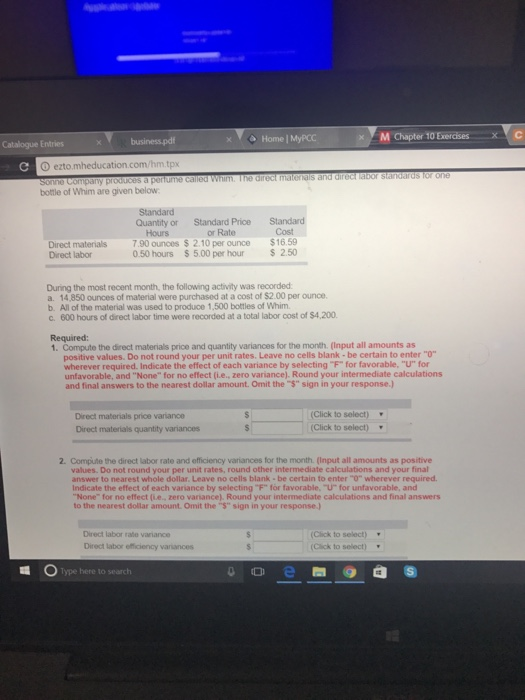# Question & Answer: Sonne Company produces a perfume called Whim. The direct materials and direct la…..Don't use plagiarized sources. Get Your Custom Essay on
Question & Answer: Sonne Company produces a perfume called Whim. The direct materials and direct la…..
GET AN ESSAY WRITTEN FOR YOU FROM AS LOW AS \$13/PAGE

Direct Materials Price Variance:

Actual Quantity = 14,850 ounces
Actual Rate = \$2.00 per ounce
Standard Rate = \$2.10 per ounce

Direct Materials Price Variance = (Actual Rate – Standard Rate)*Actual Quantity
Direct Materials Price Variance = (\$2.00 – \$2.10)*14,850
Direct Materials Price Variance = \$1,485 Favorable

Direct Material Quantity Variance:

Actual Quantity = 14,850 ounce
Standard Rate = \$2.10 per ounce

Standard Quantity = 7.90 ounces per unit * 1,500 unit
Standard Quantity = 11,850 ounces

Direct Material Quantity Variance = (Actual Quantity – Standard Quantity)*Standard Rate
Direct Material Quantity Variance = (14,850 – 11,850) * \$2.10
Direct Material Quantity Variance = \$6,300 Unfavorable

Direct Labor Rate Variance:

Actual Hours = 600
Actual Cost = \$4,200
Standard Rate = \$5.00 per hour

Direct Labor Price Variance = Actual Cost – Standard Rate*Actual Quantity
Direct Labor Price Variance = \$4,200 – \$5.00*600
Direct Labor Price Variance = \$1,200 Unfavorable

Direct Labor Efficiency Variance:

Actual hours = 600
Standard Rate = \$5.00 per hour

Standard hours = 0.50 hours per unit * 1,500 unit
Standard hours = 750

Direct Labor Efficiency Variance = (Actual Hours – Standard hours)*Standard Rate
Direct Labor Efficiency Variance = (600 – 750) *\$5.00
Direct Labor Efficiency Variance = \$750 Favorable